# Quadrilateral Shapes List & Flashcards

1/23 (missed) 0 0
Create Your Account To Continue Studying

As a member, you'll also get unlimited access to over 79,000 lessons in math, English, science, history, and more. Plus, get practice tests, quizzes, and personalized coaching to help you succeed.

Try it risk-free for 30 days. Cancel anytime
Identify if the figure is convex or concave: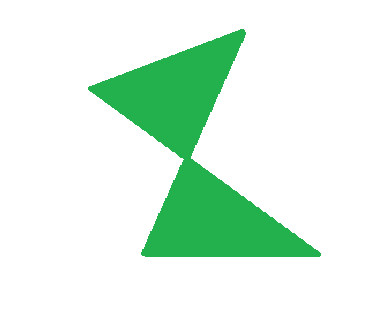Concave
Got it
Identify if the figure is convex or concave: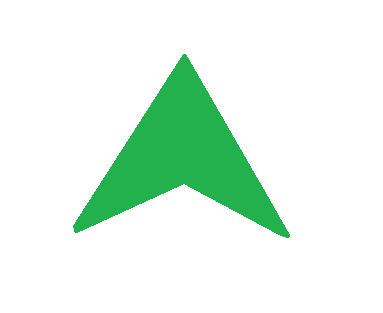Concave
Got it
If one of the line segments that are created by connecting opposite vertices lies outside of the quadrilateral, the figure is concave.
Got it
If the line segments that are created by connecting opposite vertices lie within the quadrilateral, the figure is convex.
Got it
Vertex
Where two sides of a figure connect. Always labeled with a capital letter.
Got it
A two dimensional closed figure with four sides.
Got it
Identify the quadrilateral given: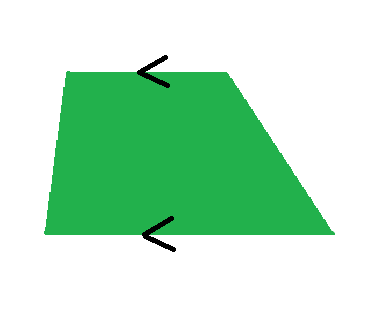Trapezoid
Got it
Identify the quadrilateral given: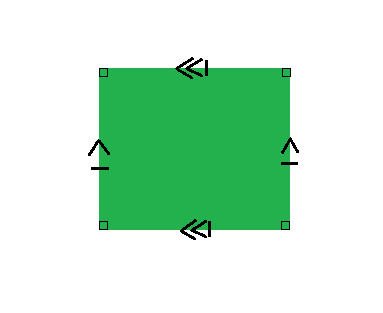Square
Got it
Identify the quadrilateral given: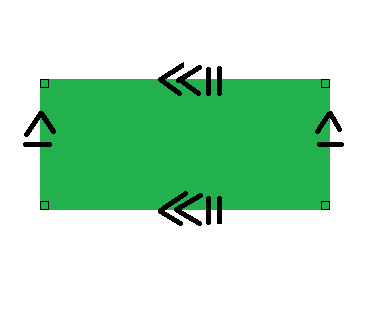Rectangle
Got it
Identify the quadrilateral given: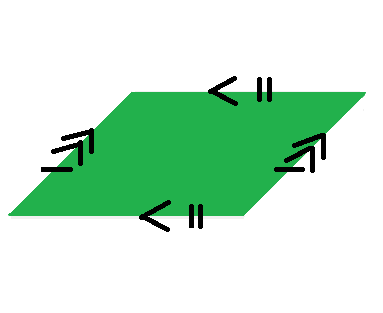Parallelogram
Got it
Identify the quadrilateral given: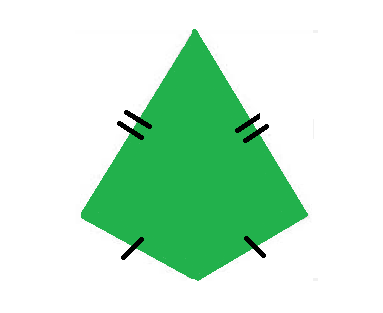Kite
Got it

Go to next set in chapter: Next set in chapter Shapes Flashcards
or choose a specific lesson: See all lessons in this chapter
23 cards in set

## Flashcard Content Overview

While many shapes are given their own specific name, they can be grouped under a broader category based on similar properties they share with other figures. Non-regular shapes that have no name can be categorized using the same method. This flashcard set focuses on the broader shape heading of 'quadrilaterals'. Do you know what the shared characteristics of quadrilaterals are? Can you identify if they are convex or concave? Use this flashcard set to test your knowledge of quadrilaterals and the shapes that are under this title.

## Studying with Flashcards

We are used to calling shapes by their common name (i.e. rectangle, square, etc.), but we have to remember that many shapes can be classified in more than one way. Think of this as a way to group shapes by common properties so there is less that you have to know in order to classify each one. For example, what if you are asked to identify a shape but the interior angle measures and side lengths are unknown? Is it a rectangle? A parallelogram? After studying this set you will see that labeling it as a quadrilateral takes away the need to know the additional measurements. Use this flashcard set as a way to master the various shapes that fall under the quadrilateral heading or to run through it quickly before a test. Either way, it can provide you with a quick reference when needed.

Front
BackKiteParallelogramRectangleSquareTrapezoid
A two dimensional closed figure with four sides.
Vertex
Where two sides of a figure connect. Always labeled with a capital letter.
If the line segments that are created by connecting opposite vertices lie within the quadrilateral, the figure is convex.
If one of the line segments that are created by connecting opposite vertices lies outside of the quadrilateral, the figure is concave.
Identify if the figure is convex or concave:Concave
Identify if the figure is convex or concave:Concave
Identify if the figure is convex or concave: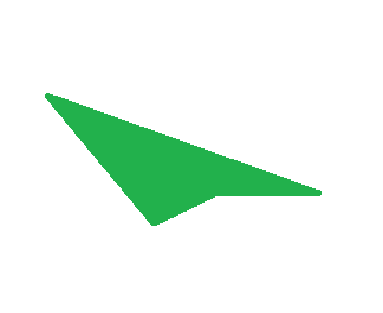Concave
Identify if the figure is convex or concave: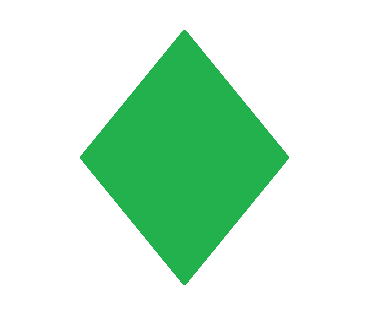Convex
Identify if the figure is convex or concave: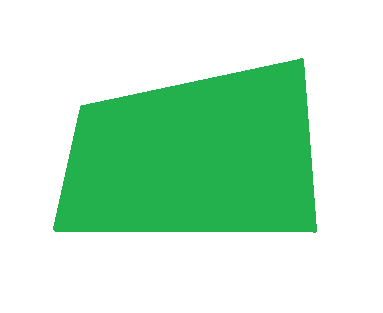Convex
Identify if the figure is convex or concave: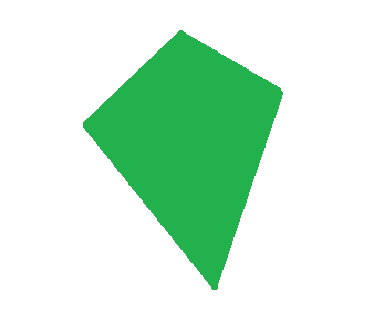Convex
Square
A quadrilateral with four right angles and four sides that are equal in length.
Rectangle
A quadrilateral with four right angles and opposite sides equal in length.
Parallelogram
A quadrilateral with opposite sides that are equal in length and that are parallel.
Rhombus
A quadrilateral with four sides that are equal in length but do not have four right interior angles.
Kite
A quadrilateral with two pairs of adjacent sides that are equal in length.
Trapezoid
A quadrilateral with at least one pair of parallel sides.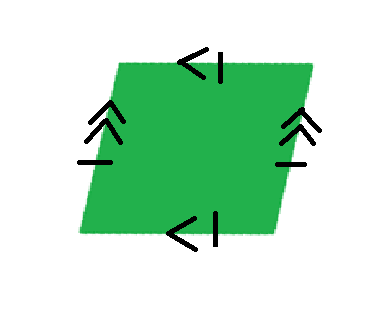Rhombus
Fill in the correct degree amount: the sum of the interior angles of a quadrilateral is _____.
360o

To unlock this flashcard set you must be a Study.com Member.